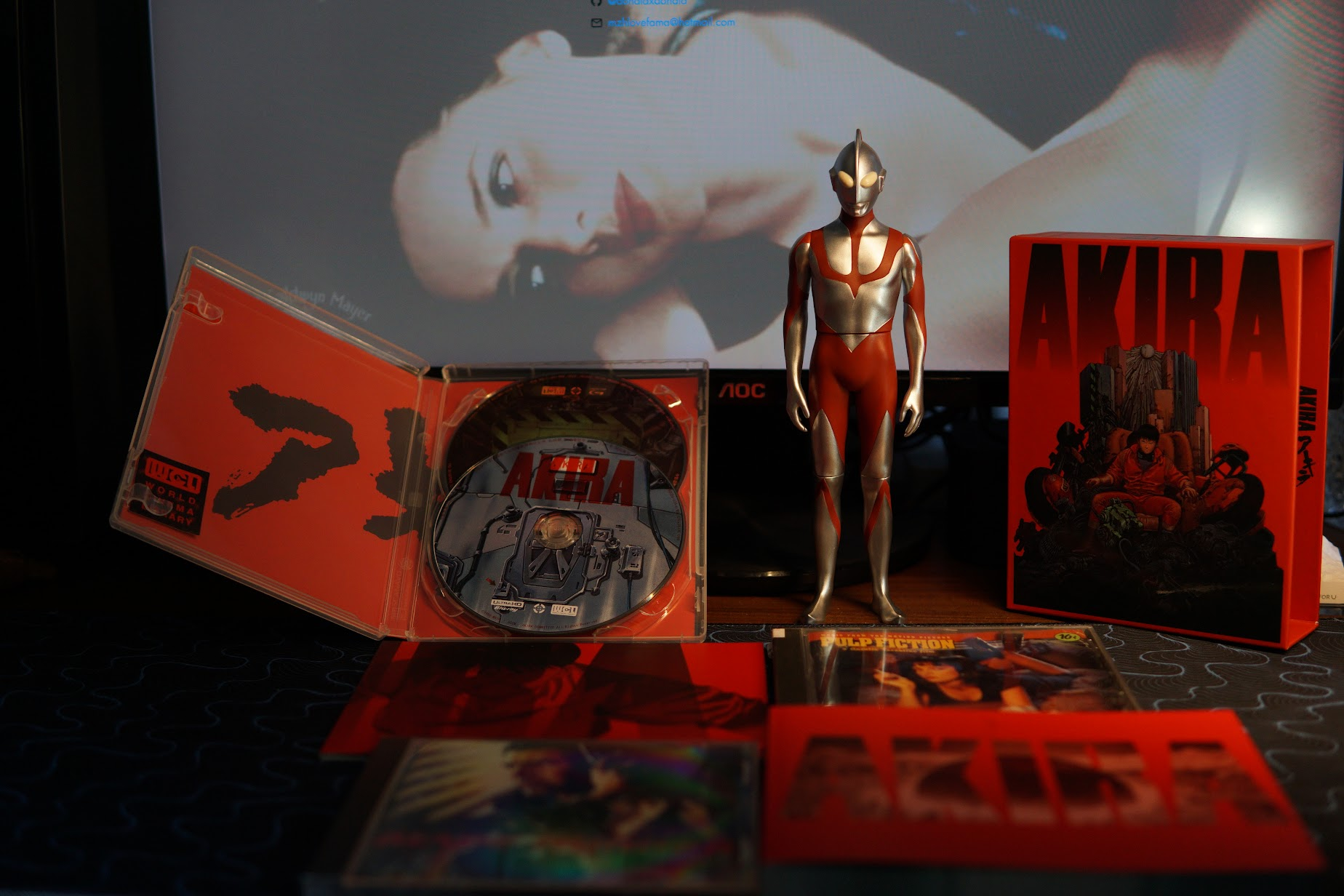# State of 2021self.__wrap_n=self.__wrap_n||(self.CSS&&CSS.supports("text-wrap","balance")?1:2);self.__wrap_b=(e,n,t)=>{t=t||document.querySelector(`[data-br="\${e}"]`);let r=t.parentElement,o=f=>t.style.maxWidth=f+"px";t.style.maxWidth="";let c=r.clientWidth,a=r.clientHeight,s=c/2-.25,i=c+.5,p;if(c){for(o(s),s=Math.max(t.scrollWidth,s);s+1<i;)p=Math.round((s+i)/2),o(p),r.clientHeight===a?i=p:s=p;o(i*n+c*(1-n))}t.__wrap_o||(typeof ResizeObserver!="undefined"?(t.__wrap_o=new ResizeObserver(()=>{self.__wrap_b(0,+t.dataset.brr,t)})).observe(r): false&&0)};self.__wrap_n!=1&&self.__wrap_b(":Rarbb9mba:",1)

## #春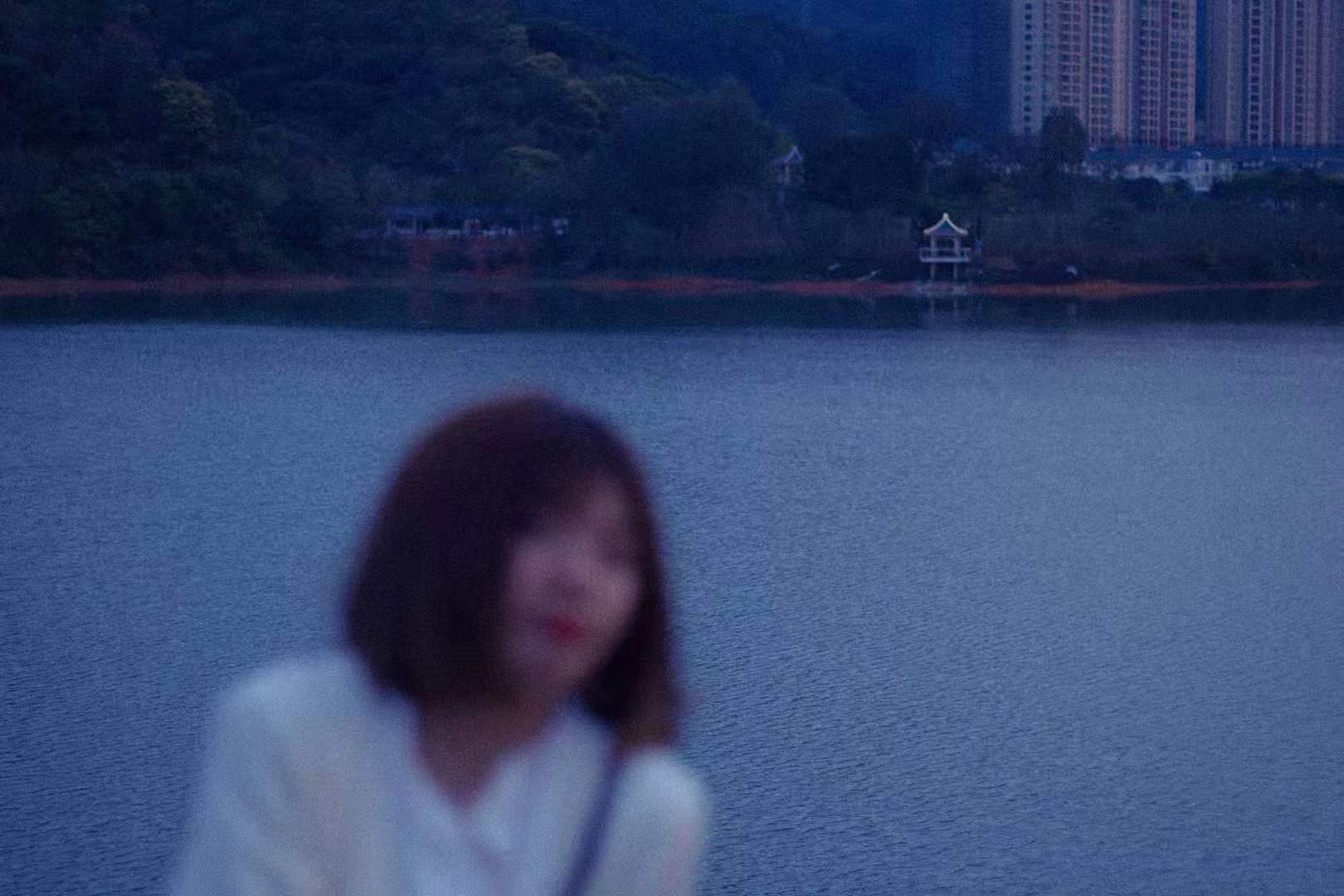2021 年里比较大的一个点可以说是和 Florence 互相见家长了，也是我们在一起八年以来第一次和彼此父母坦白自己的感情状况，毕竟在学生时代没啥经济能力的时候见家长也不太好，免得听他们说这说那。Florence 的爸妈都挺 nice 的，每次去都对我很热情，但社恐的我真的只想在房间里静静地呆着。

## #夏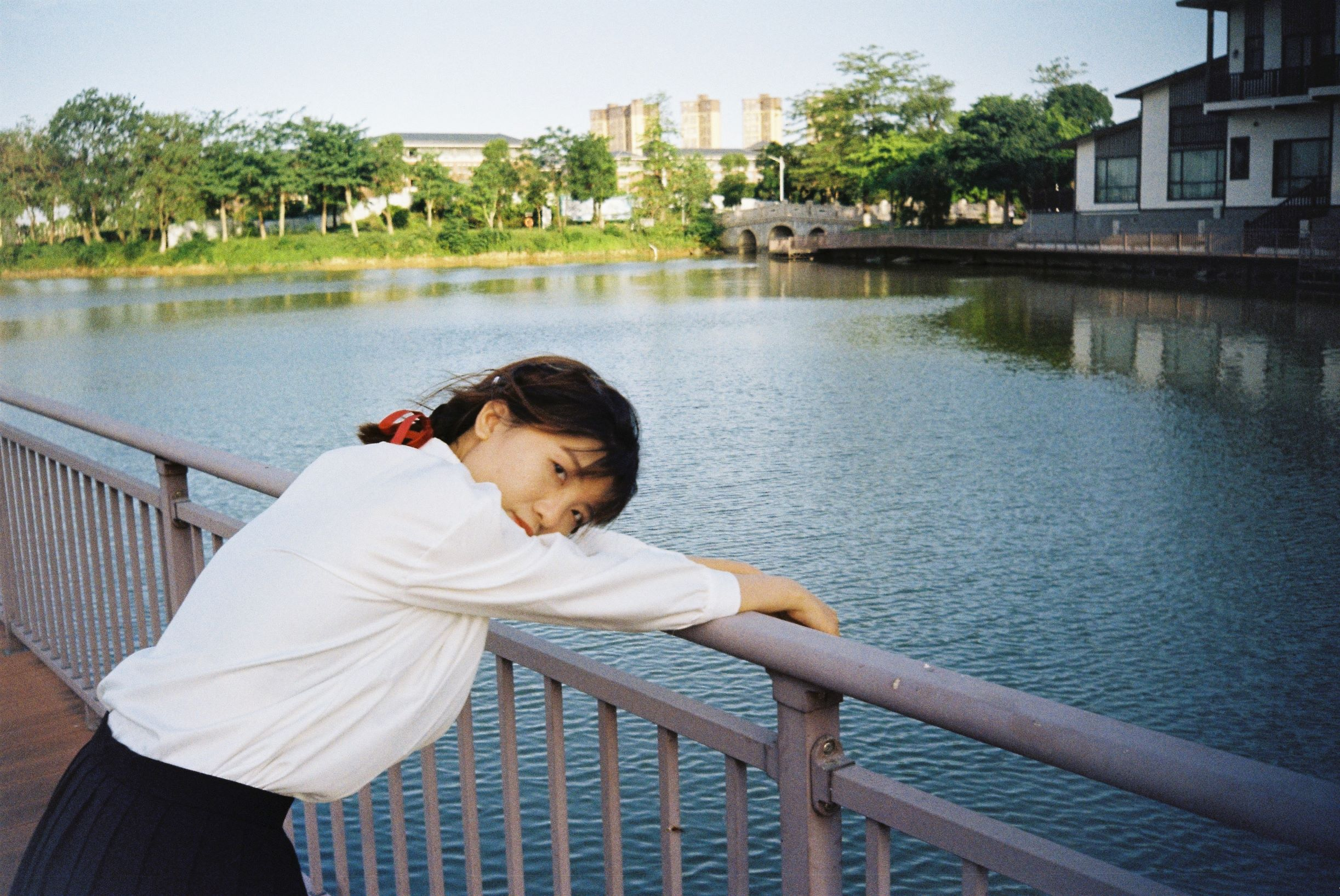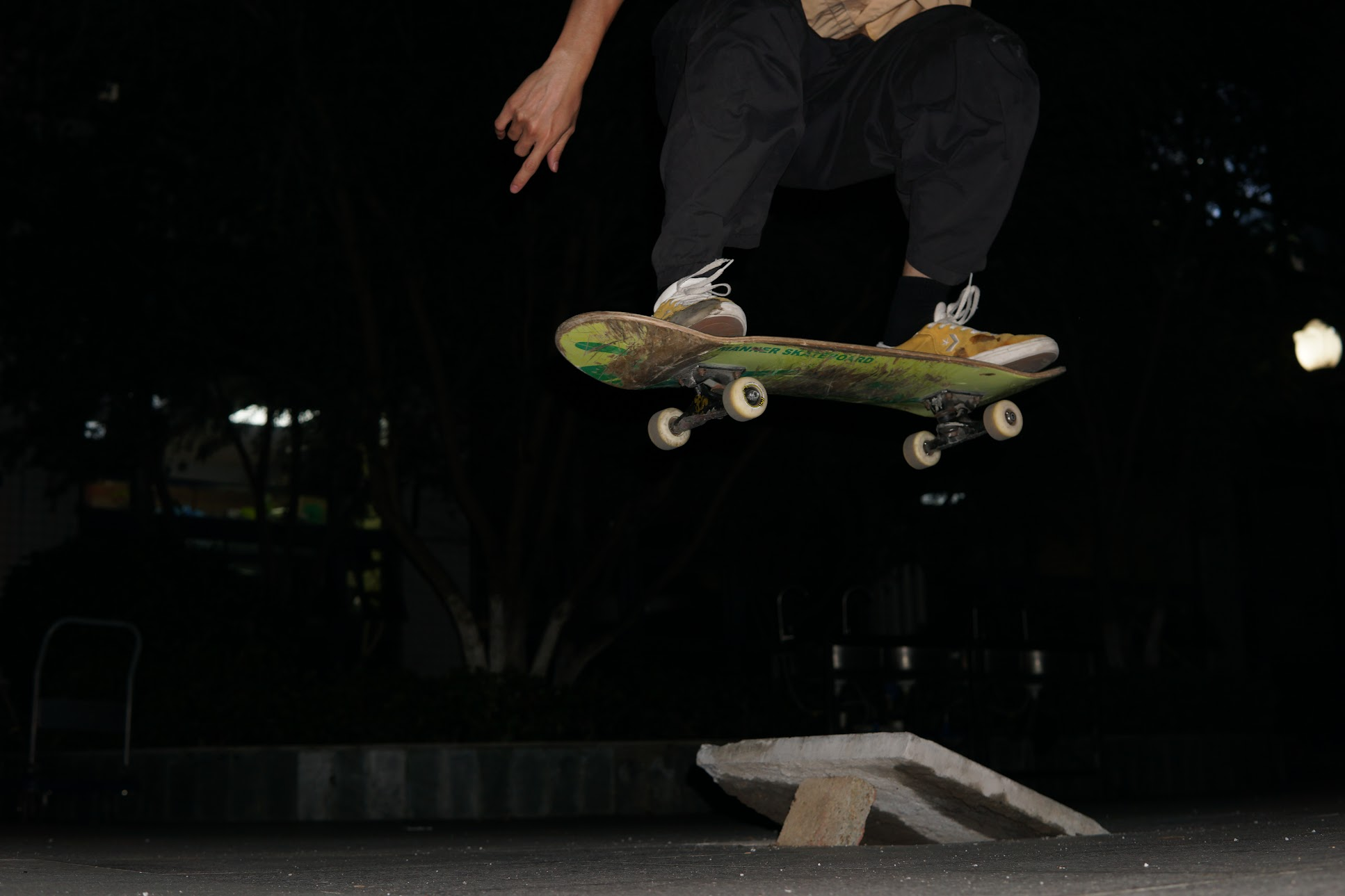## #秋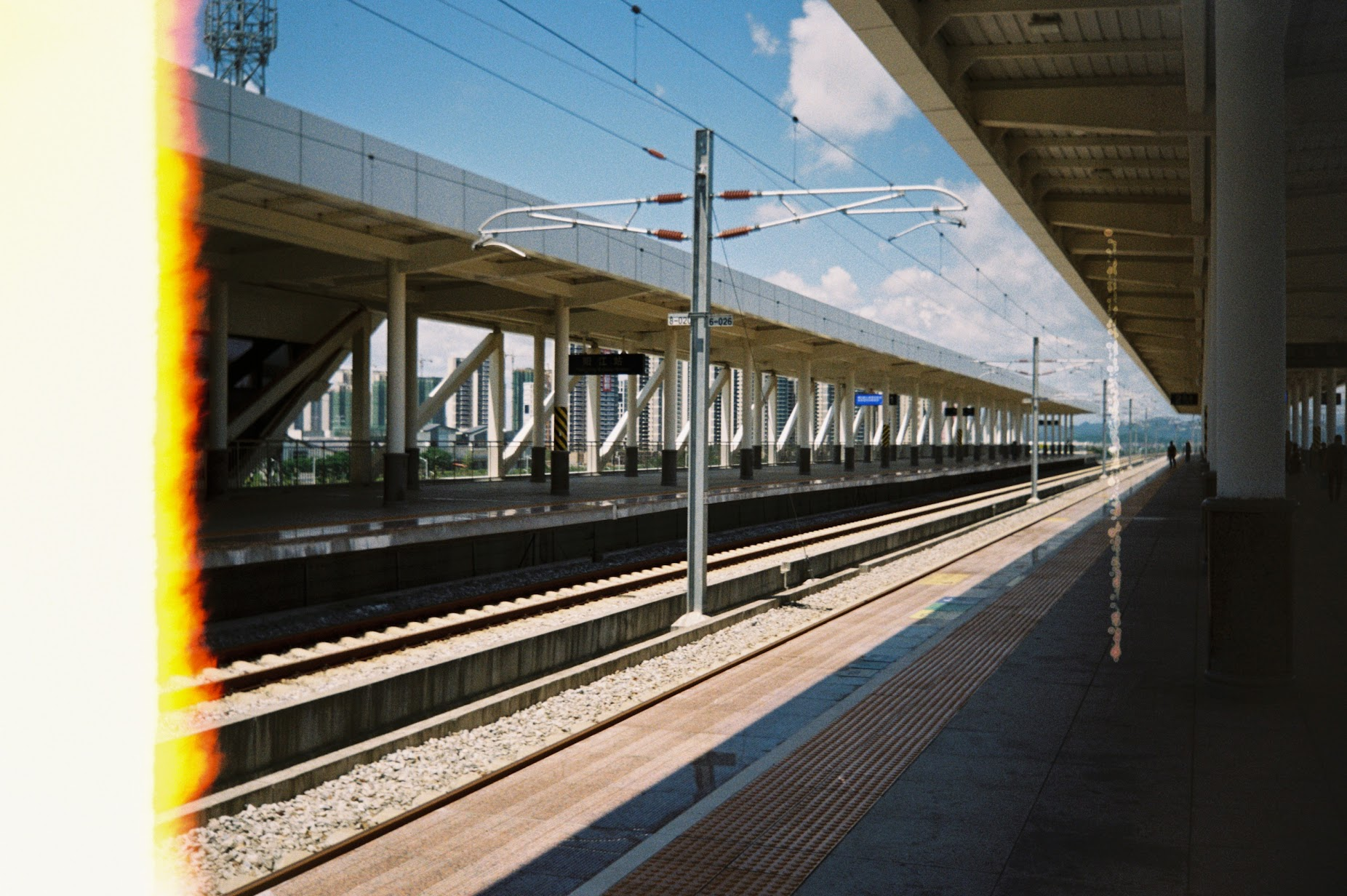## #冬